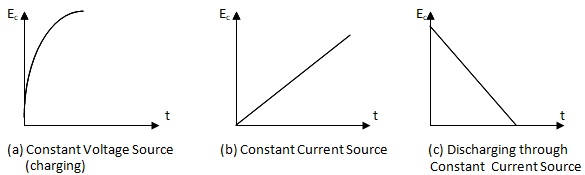### Digital Voltmeter - Advantages, Features, Principle

A digital voltmeter is a voltage measuring device. It indicates the voltage under measurement in discrete numerals. It is an electronic voltmeter. It mostly uses integrated circuits for its functioning. The numerical display is either by LEDs or LCDs.

### (a) Advantages of Digital Voltmeter:

The following are the advantages of digital Voltmeter:

1. There will be lot of saving of the reading time.
2. Interpolation errors are reduced.
3. Parallax error is avoided.
4. The digital readout is cheaper.
5. They offer good resolution.
6. They are versatile.
7. They are adaptable.

### (b) The Outstanding Features of Digital Voltmeters (Digital Multimeters):

The outstanding features of digital voltmeters or in general digital multimeters are listed below.

1. The digital voltmeters (multimeters) are compact in size. They are very accurate. Their small size makes them more suited for field work.
2. The input range can be from the lowest voltage of ± 1.000000 V to ± 1000.000 V.
3. They have auto range and overload indication.
4. Absolute accuracy high of the order of + 0.005% of the reading.
5. The short term stability is of the order of 0.002% of the reading over a period of 24 hours.
6. The long term stability is of 0.008% of the reading for a period of 6 months.
7. They offer a very good resolution of 1 part in 106. That is we will be able to read 1 µV in 1 V range.
8. The input impedance offered is large of the order of 10 M Ω.
9. The input capacitance is low of the order of 40 pF.
10. The calibration of the instrument is independent of the circuit under test.
11. The readings displayed can be recorded, digitally processed and printed results can be obtained by providing necessary adaptors.
12. The digital voltmeters are capable of measuring resistance, direct current, power, alternating voltage alternating current and steady voltage (D.C. volts). They can be used to test electronic devices. They can be used to measure physical variables with suitable transducers. These measurements require additional switching circuit with basic digital voltmeter module.

### (c) Classification of Digital Voltmeters (D.V.M.):

They are classified in to the following types

(i) Ramp type.
(ii) Integrating type.
(iii) Continuous balance type.
(iv) Successive approximation type.

### (d) Principle of Ramp Type Digital Voltmeter

The basic principle of the ramp type of digital voltmeter is to convert the unknown voltage to time. Time has been chosen to utilise the simple digital counters, and an oscillator of known frequency to display the time in a digital format.

The unknown voltage is to be converted in to time linearly. This is done by charging a capacitor through a resistance. We know that when a voltage is applied to such an R.C. circuit the voltage across the capacitor increases with time. The voltage reaches the applied voltage after the capacitor has completely charged.

It is to be noted here that the charging curve of the capacitor is not linear. In order to obtain a linear charge we have to charge the capacitor using a constant current source instead of a constant voltage source. The capacitor can be discharged through the constant current source. Therefore by charging the capacitor with the voltage to be measured we are converting the voltage to a time varying voltage across the capacitor.

As the charging curve is having its slope depending on the value of capacitance and resistance used for charging, this method of converting analog signal to digital signal is called ramp or slop conversion.

### (e) The Types of Slope Conversion:

There are two methods of the slope conversion.

1. The single slope conversion and
2. The dual slope conversion.Charging and Discharging curves of Capacitor
Using comparators, counter and the associated circuitry, digital display of the applied voltage can be obtained. The charging curves of a capacitor using constant voltage source and constant current source are shown in Figure. The discharge curve through the constant current source is shown in the same figure.